# Cosine Function

Cosine Function

The cosine function is one of the primary mathematical trigonometric functions. It is complementary to the cosine function (co+cosine). Cosine function was first used in ancient Egypt in the book of Ahmes (c. 2000 B.C.). Much later F. Viète (1590) evaluated some values, E. Gunter (1636) introduced the notation "Cosi" and the word "cosinus" (replacing "complementi sinus"), and I. Newton (1658, 1665) found the series expansion for cosine.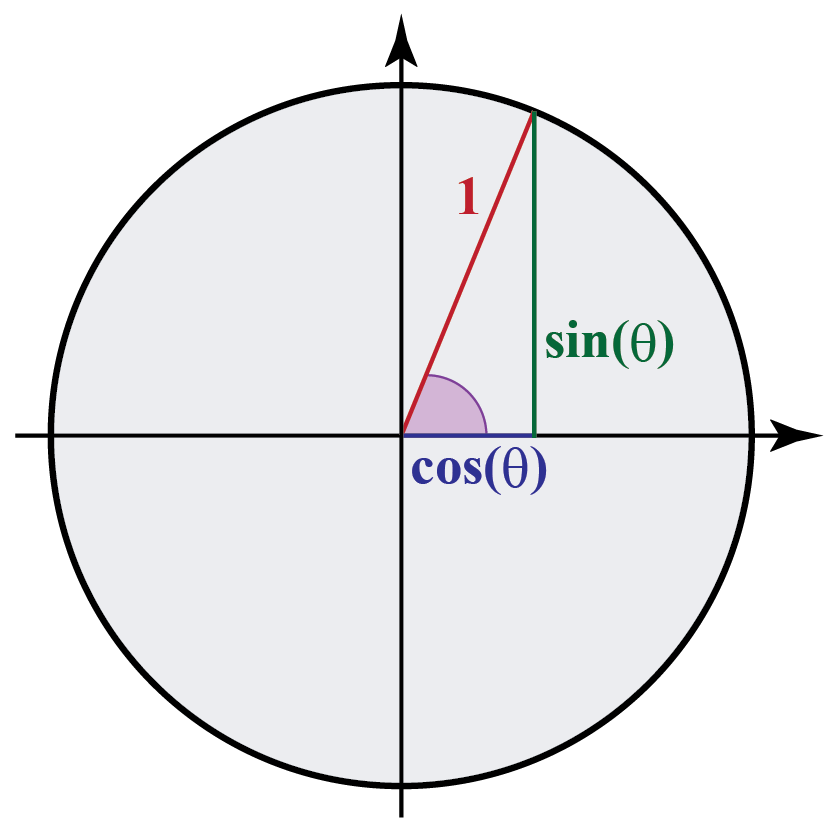In this mini-lesson, we will explore the world of the cosine function. The journey will take us through cosine function, unit circle, and angles, how to use them, and their various applications. You can check out the interactive calculator to know more about the lesson and try your hand at solving a few interesting practice questions at the end of the page.

## Lesson Plan

 1 What Is a Cosine Function? 2 Important Notes on Cosine Function 3 Solved Examples on Cosine Function 4 Interactive Questions on Cosine Function 5 Thinking Out of the Box!

## What Is a Cosine Function?

The cosine function is a periodic function in trigonometry.

The cosine function or cos function can be defined as the ratio of the length of the base to that of the length of the hypotenuse in a right-angled triangle.

Let us try to understand the concept of cosine function by analyzing the four quadrants of the coordinate axis system.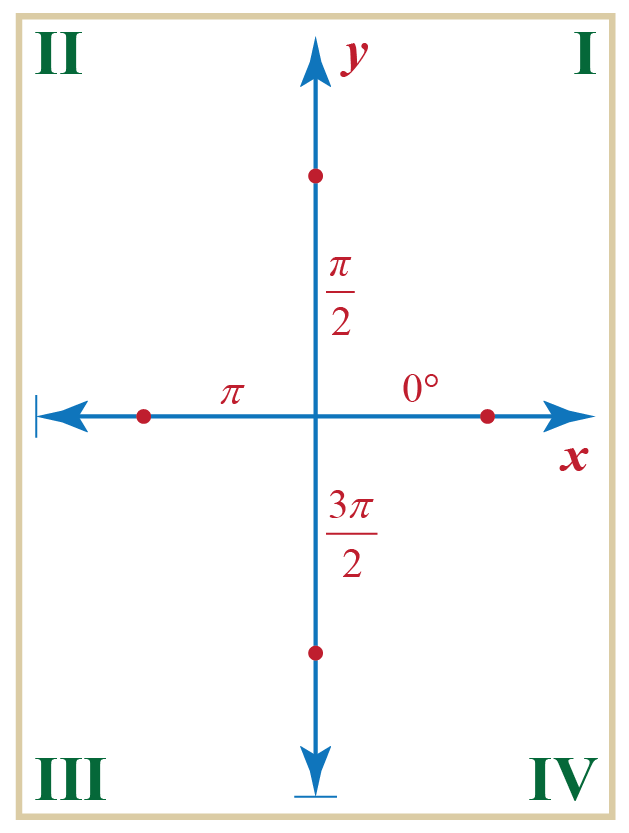Now, consider a unit circle centered at the origin of the coordinate plane.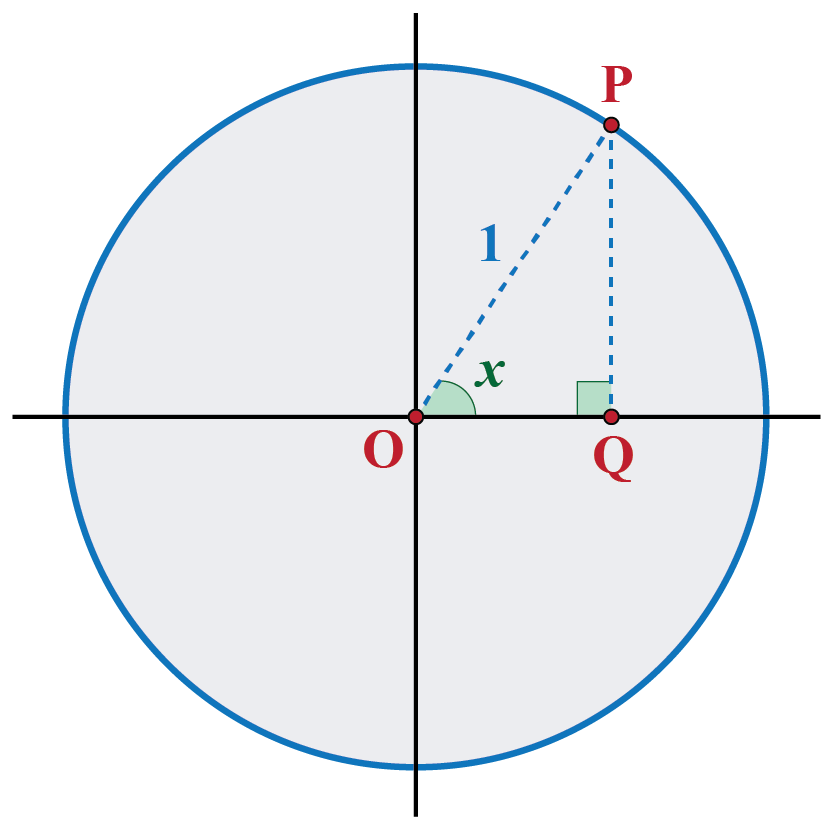A variable point $$P$$ is taken on the circumference of the circle and it continues to moves on the circumference of this circle.

From the figure, we observe that $$P$$ is in the first quadrant, and $$OP$$ makes an acute angle of $$x$$ radians with the positive $$x$$-axis.

$$PQ$$ is the perpendicular dropped from $$P$$(a point on the circumference) on to the x-axis.

The triangle is thus formed by joining the points O, P, and Q as shown in the figure, where OQ is the base, and PQ is the height of the triangle.

Hence, the cosine function or cos function for the above case can be mathematically written as:

 $$\cos x = \frac{{OQ}}{{OP}}$$

Here, x is the acute angle formed between the hypotenuse and the base of a right-angled triangle.

## Cosine Graph

The following figure shows a unit circle with center $$O$$ at the origin, and a point $$P$$ moving along the circumference of this circle.

The angle which $$OP$$ makes with the positive direction of the $$x$$-axis is $$x$$ (radians).

$$PQ$$ is the base dropped from $$P$$ to the horizontal axis.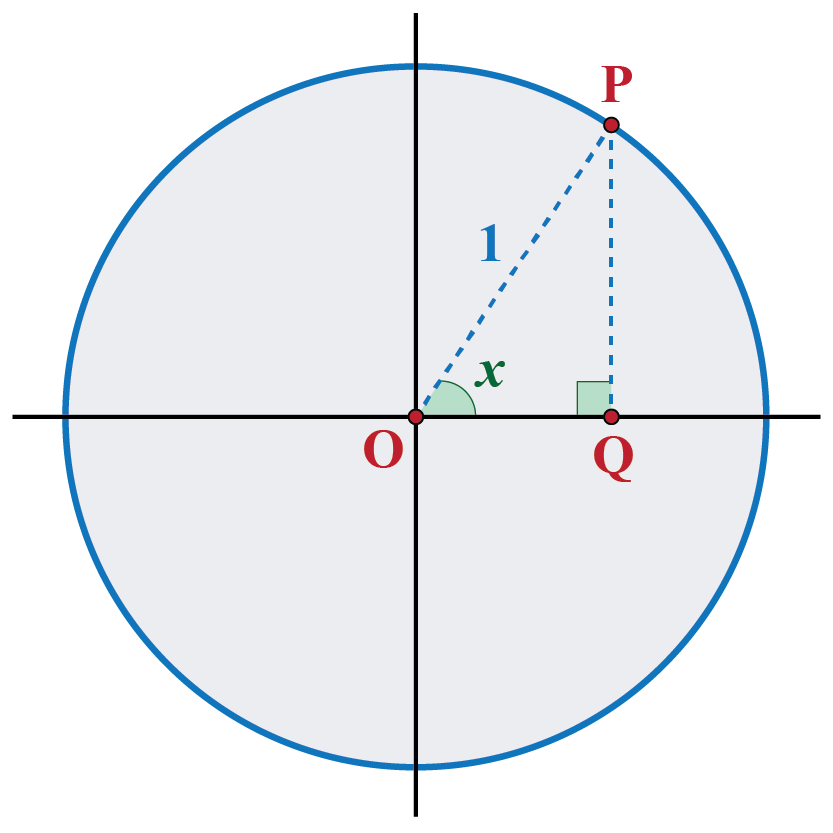We note that: $\cos x = \frac{{OQ}}{{OP}} = \frac{{OQ}}{1} = OQ$

As $$x$$ varies, we study that:

The value of $$\cos x$$ varies with the variation of the length of $$OQ$$.

Case 1: Variation of OQ in the first quadrant.

Suppose that initially, $$P$$ is on the horizontal axis. Let us consider a movement of $$P$$ through $$90^\circ$$ or $$\frac{\pi }{2}$$ rad.

The following figure shows different positions of $$P$$ for this movement: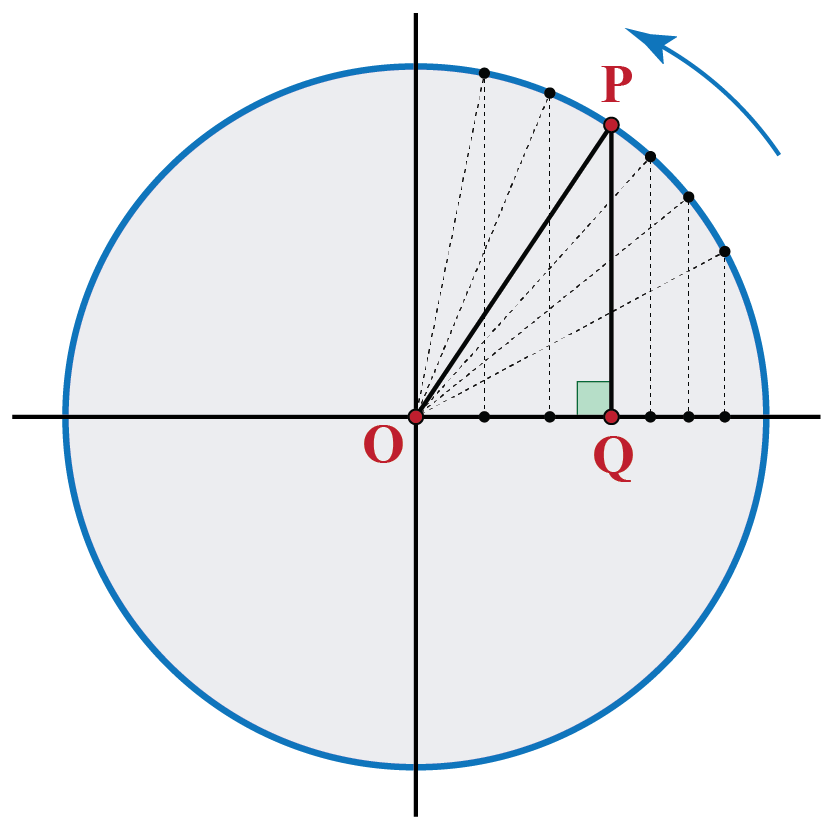Clearly, $$OQ$$ has decreased in length, from an initial value of 1 (when $$x$$ is 0 radians) to a final value of 0 (when $$x$$ is $$\frac{\pi }{2}$$ radians).

We can now plot this variation.

The horizontal axis represents the input variable $$x$$ is the angle in radians, and the vertical axis represents the value of the cosine function for $$x$$.

The plot thus obtained is shown below: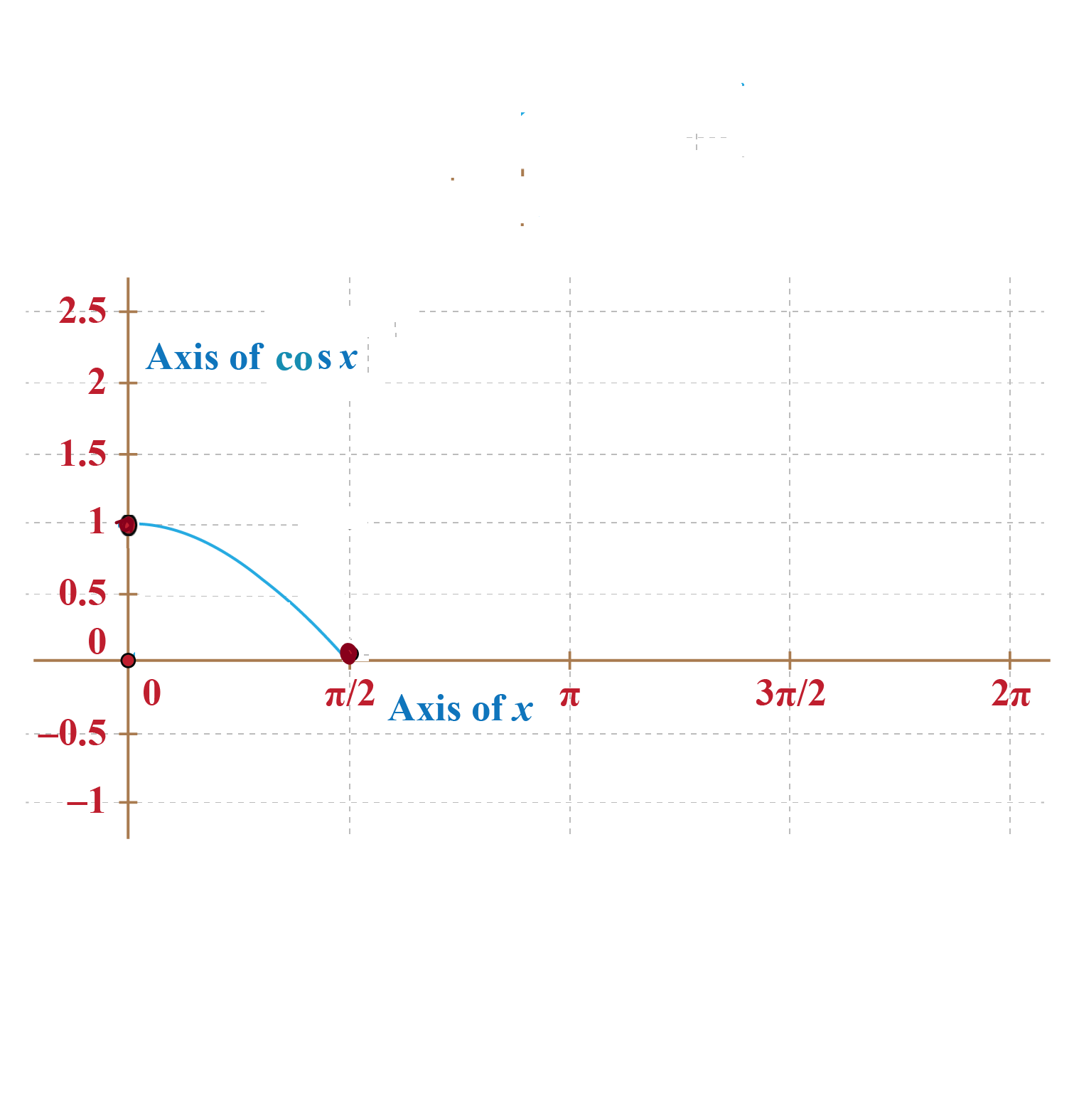Case 2: Variation of OQ in the second quadrant.

Now, let us see what happens as $$P$$ moves further.

The following figure shows different positions of $$P$$ as it subsequently moves from a $$90^\circ$$ position to $$180^\circ$$ position: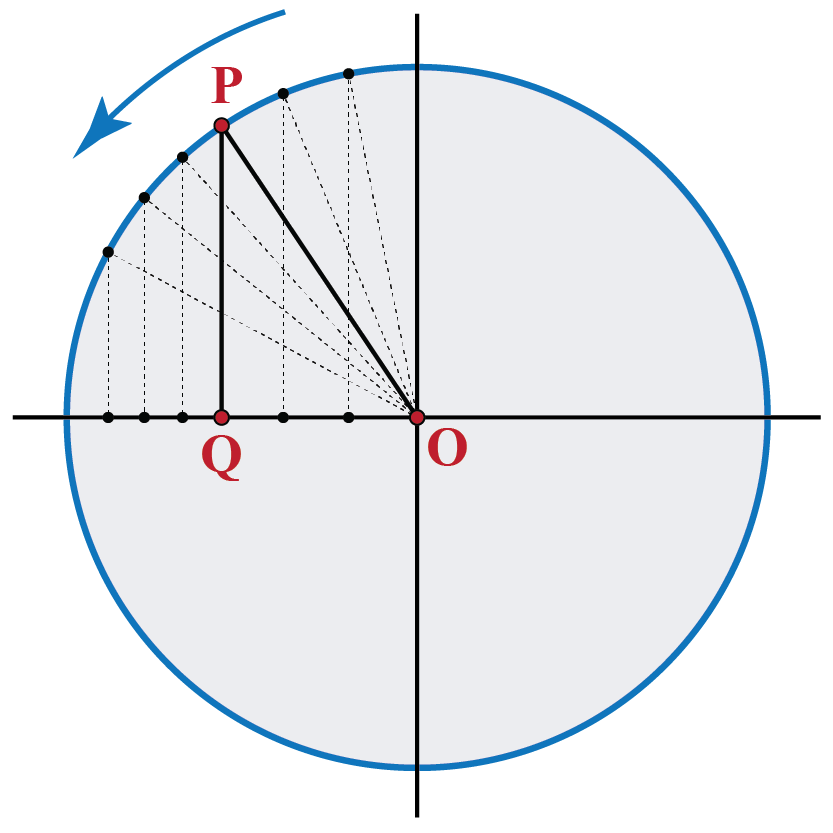In this phase of the movement, the length $$OQ$$ increases, from a minimum of 0 at $$90^\circ$$, to a maximum of 1 at $$180^\circ$$.

Though the length or magnitude of $$OQ$$ increases but the algebraic value of $$OQ$$will increase due to its direction which is along the negative y-axis.

Thus, the value of the cosine function for angle x decreases.

We continue plotting this variation on the same graph that we plotted earlier: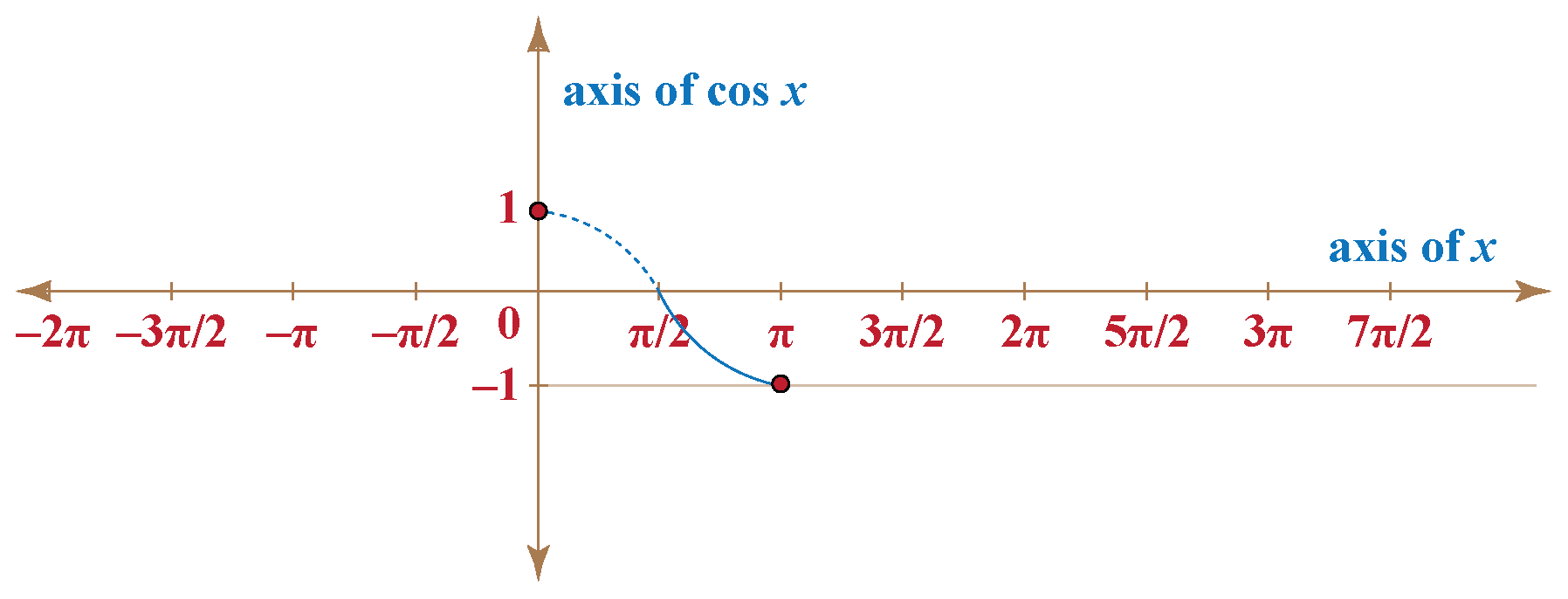Case 3: Variation of OQ in the third quadrant.

When $$P$$ moves from a position of $$180^\circ$$ to a position of $$270^\circ$$, though the length or magnitude of $$OQ$$ decreases, but since the direction is along the negative y-axis, the actual value OQ increases from -1 to 0.

Thus, the value of the cosine function for angle x increases.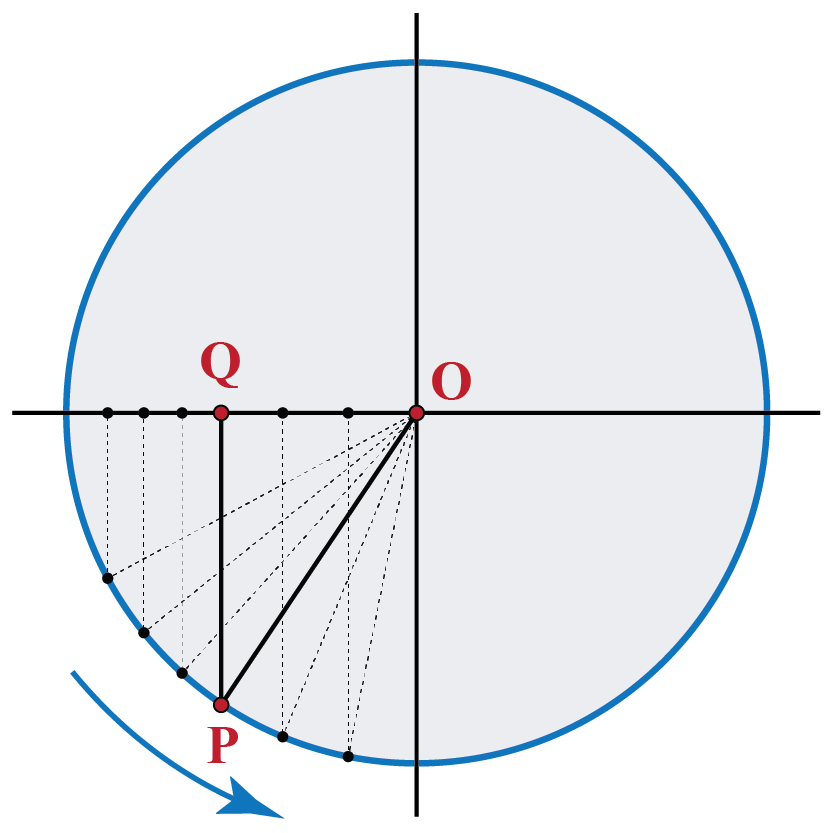We add this variation to our graph: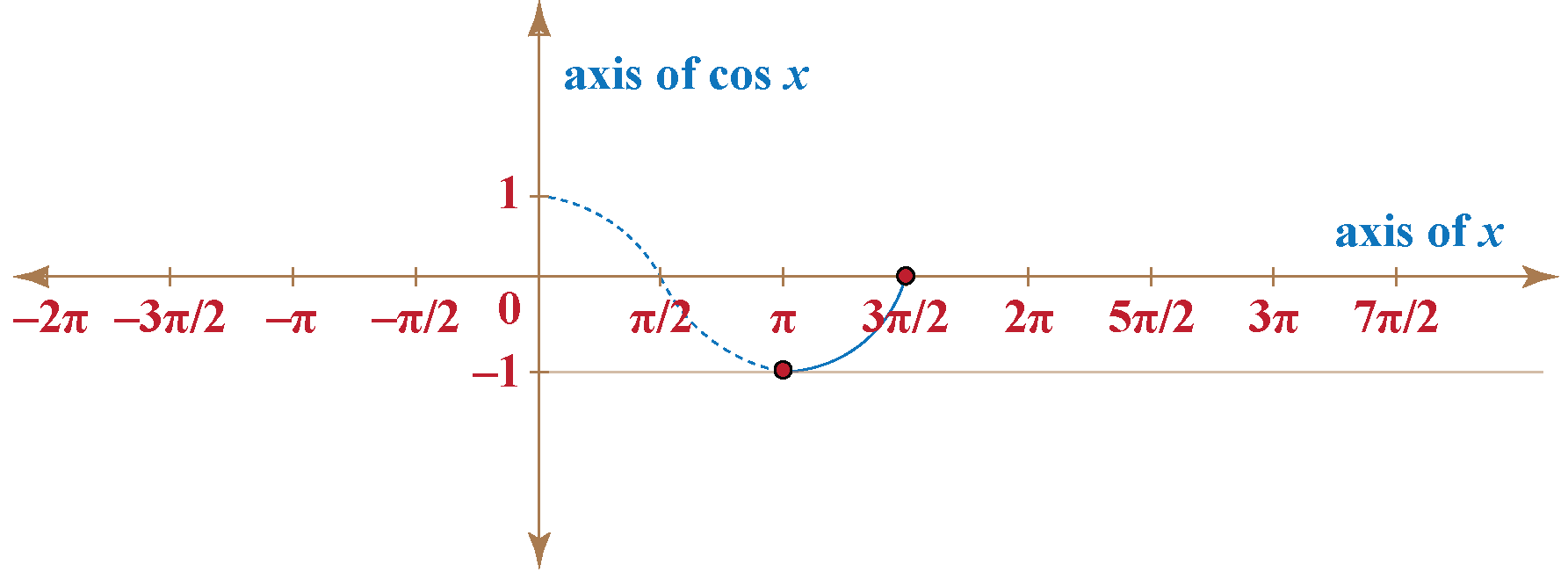Case 4: Variation of OQ in the fourth quadrant.

Finally, when $$P$$ moves from a position of $$180^\circ$$ to a position of $$360^\circ$$, $$OQ$$ increases from $$0$$ to 1.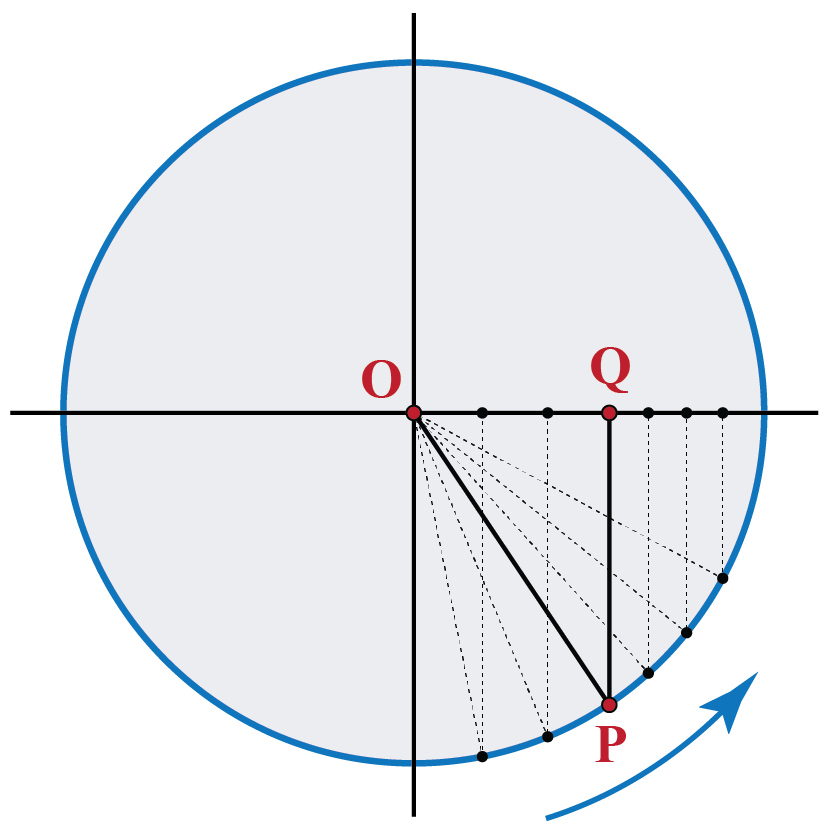The plot thus obtained is shown below: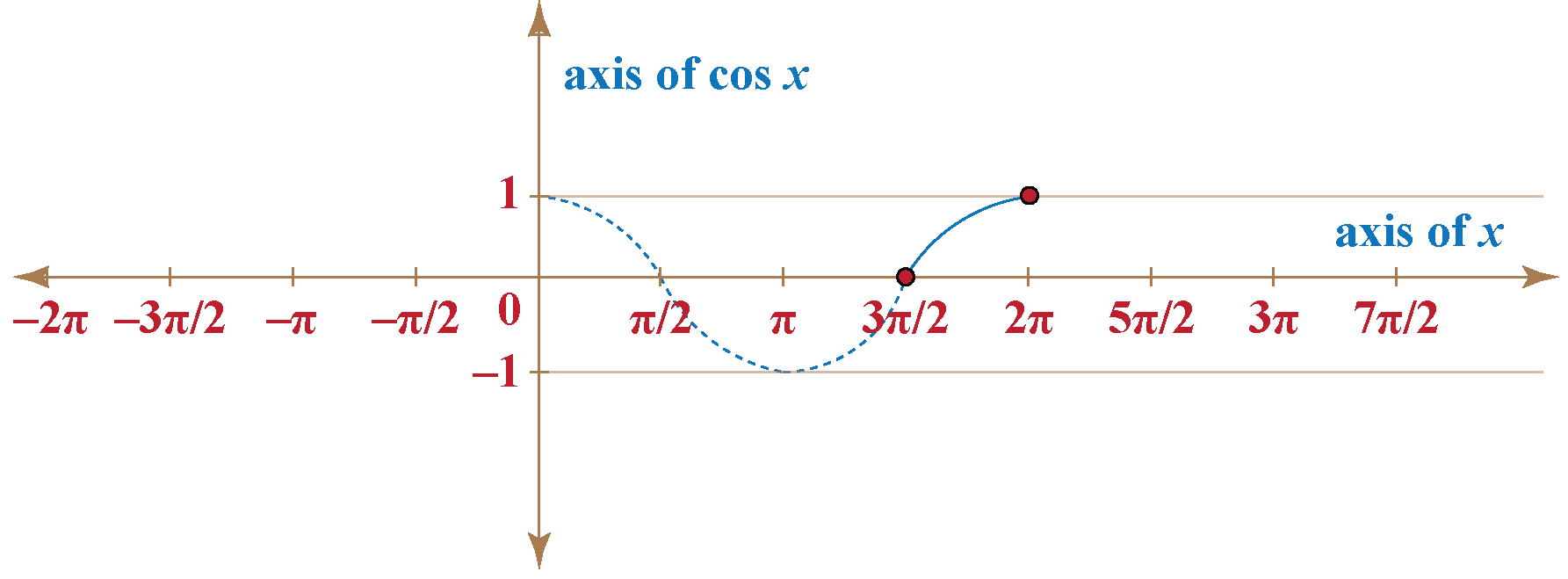Merging the response of variation in the value of $$OQ$$ for all four quadrants, we obtained the complete plot of OQ vs x or cos x vs x, for one complete cycle of $$0$$ radians to $$2 \pi$$ radians$$(0^\circ to 360^\circ)$$.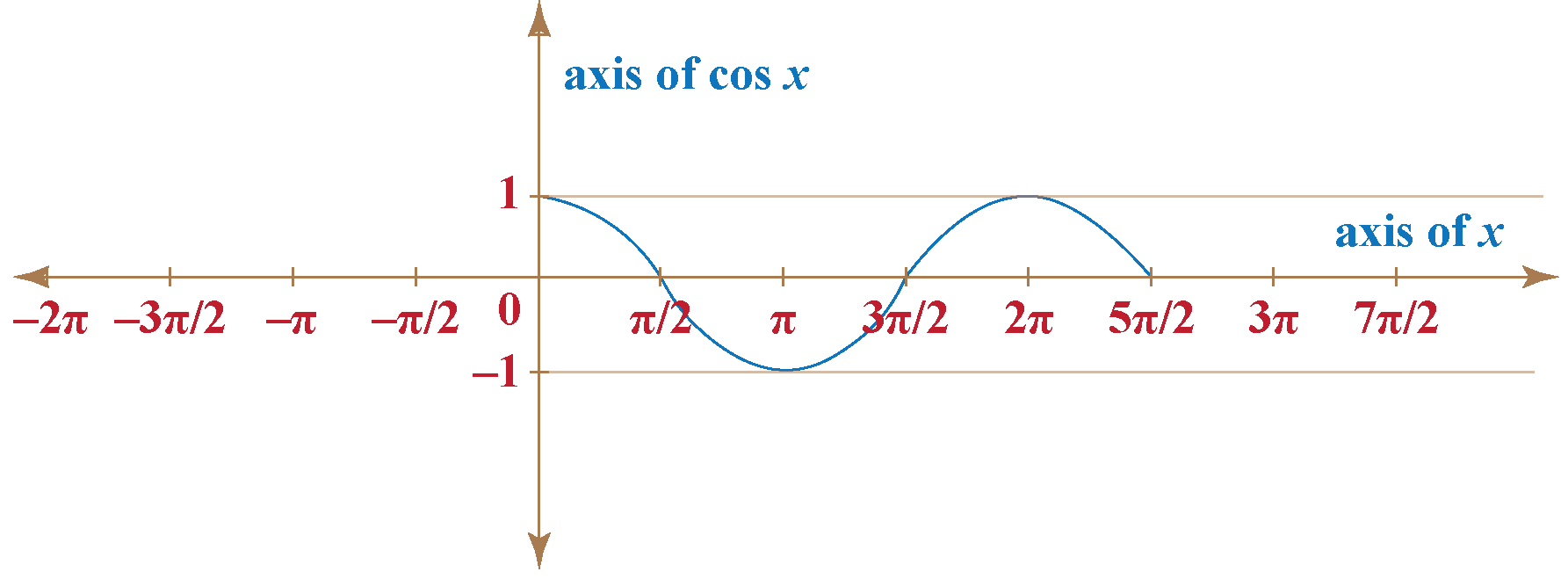What happens when $$P$$ moves further now? The same variation cycle starts all over again.

Thus, if we extend the cosine function to take on all real inputs, we obtain the following graph of cosine function: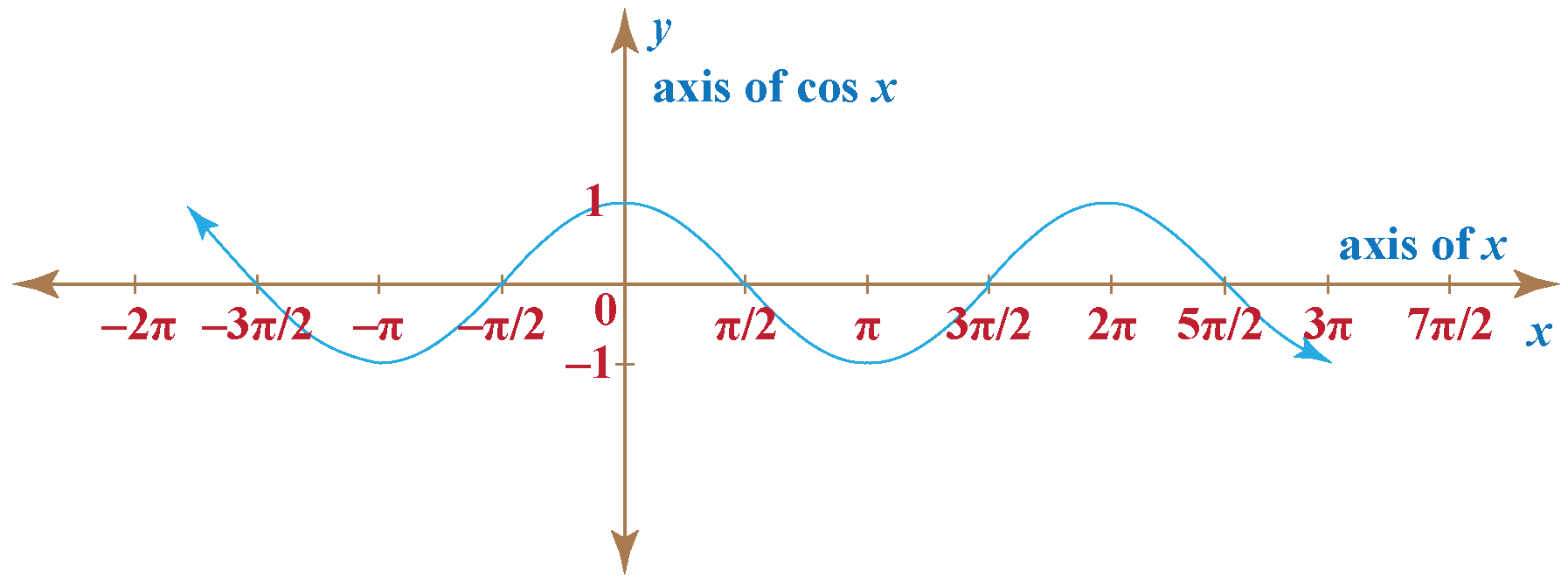## Basic Properties of Cosine Function

Properties of cosine function depend upon the quadrant in which the angle lies.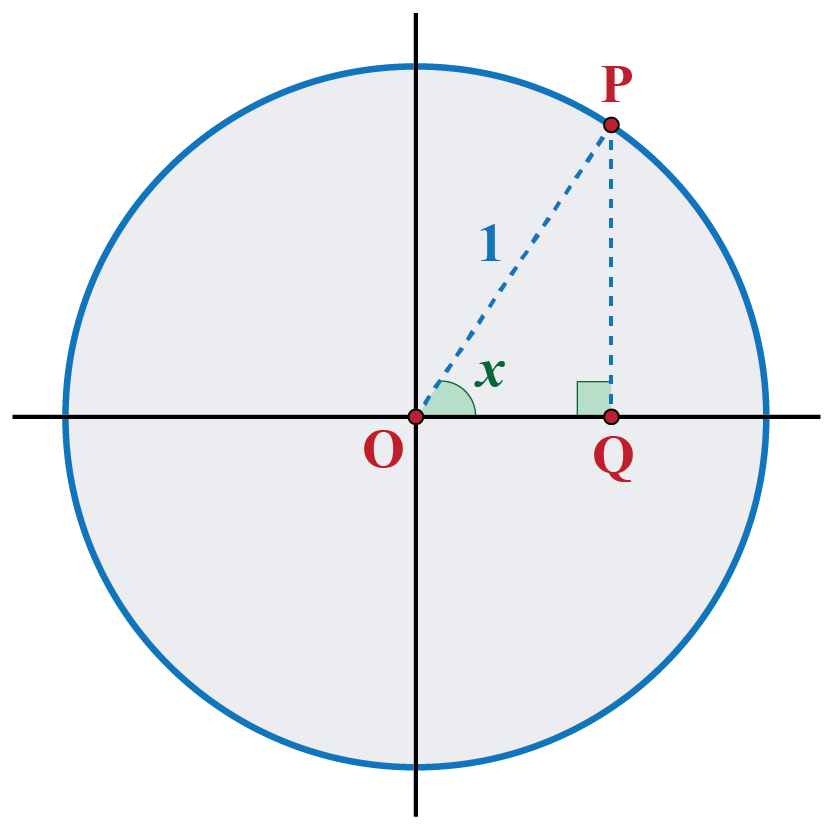From the above graph and circle relation, we can see that value of the cosine function is positive in the first and fourth quadrant whereas, in the second and third quadrant, it bears a negative value.

This can also be observed for a few values of the cosine function at different quadrants as shown in the following table of cos:

 Cosine Degrees Cosine Values cosine 0° 1 cosine 30° √3/2 cosine 45° 1/√2 cosine 60° 1/2 cosine 90° 0 cosine 120° -1/2 cosine 150° -√3/2 cosine 180° -1 cosine 270° 0 cosine 360° 1

## Range, Domain of Cosine and Other Properties

Range and Domain of Cosine Function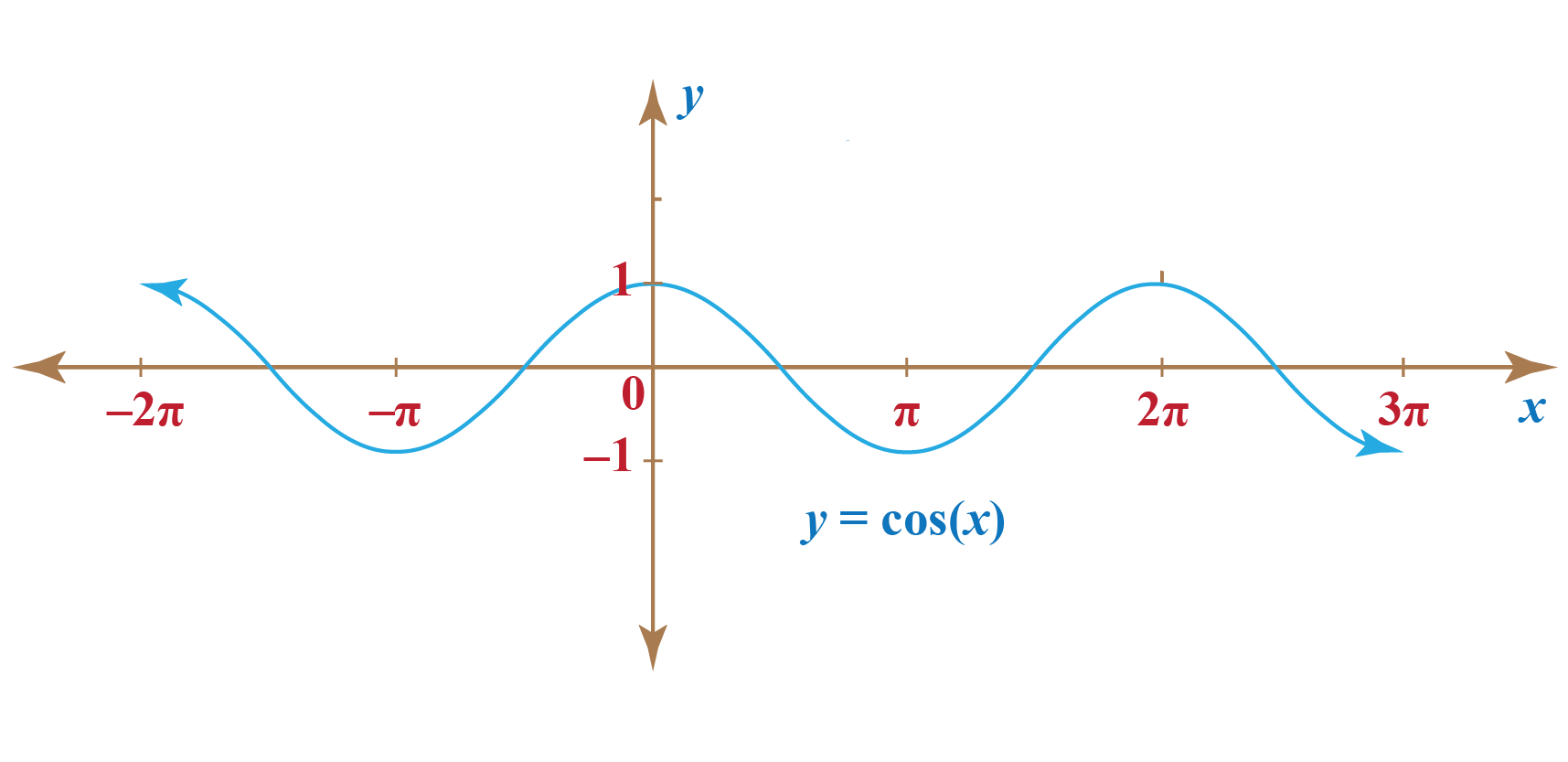Looking at the cosine function on a domain centered at the y-axis, helps us bring out its symmetry. Thus, we can see in the figure above, the cosine function is symmetric about the origin.

So the domain of cosine is all real numbers. The range of cosine is [-1,1].

Domain of cosine: R

Range of cosine: [-1,1]

Properties of Cosine Functions

• The cosine function is a periodic function
A periodic function is a function which when has a specific horizontal shift, P, results in a function equal to the original function, i.e.,
$f(x + P) = f(x)$
for all values of x within the domain of f.

The cosine graph repeats itself after 2π, which suggests the function is periodic with a period of 2π.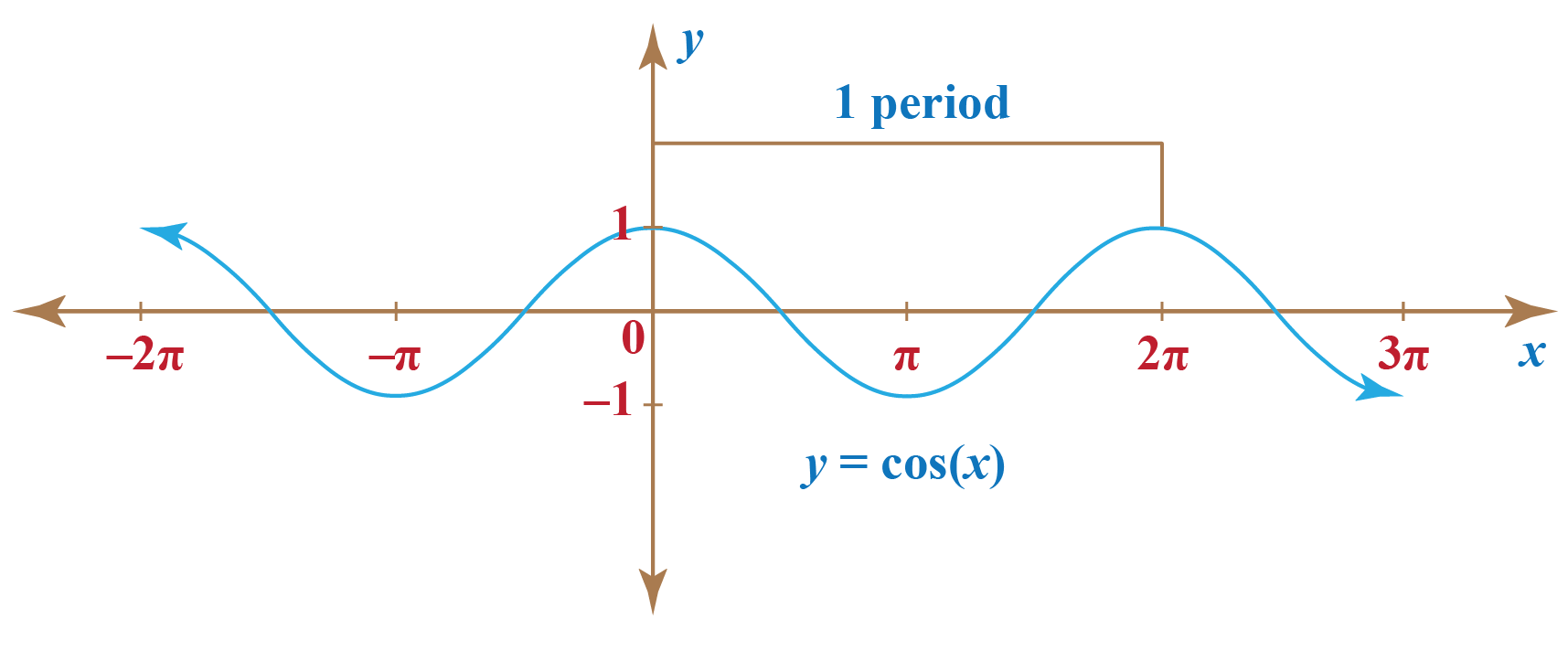So we can say that:

$\cos \left( {x + 2n\pi } \right) = \cos x\,\,\,{\rm{for \,\,\,every}}\,x$

• The cosine function is an even function:

The cosine function is an even function because $\cos(−x) = \cos x.$

## Solved Examples

 Example 1

Find the values of

a) $$\cos {780^0}$$

b) $$\cos \left( { - {{660}^0}} \right)$$

c) $$\cos \left( {\frac{{41\pi }}{4}} \right)$$

d) $$\cos \left( { - \frac{{19\pi }}{3}} \right)$$

Solution

(a) We have:

\begin{align}{780^0} &= {720^0} + {60^0}\,\,\, \\\Rightarrow \,\,\,{780^0} &\equiv {60^0}\\ \Rightarrow \,\,\,\cos \left( {{{780}^0}} \right) &= \cos \left( {{{60}^0}} \right) \\&= \dfrac{1}{2}\end{align}

 $$\therefore \ \cos {780^0} = \dfrac{1}{2}$$

(b) We note that:

\begin{align}- {660^0} &= - {720^0} + {60^0}\\ \Rightarrow - {660^0} &\equiv {60^0}\\ \Rightarrow \cos \left( { - {{660}^0}} \right) &= \cos \left( {{{60}^0}} \right)\\ &= \frac{1}{2}\end{align}

 $$\therefore \ \cos \left( { - {{660}^0}} \right) = \frac{1}{2}$$

(c) We have:

\begin{align*} \frac{{41\pi }}{4} &= 10\pi + \frac{\pi }{4}\,\,\, \\ \Rightarrow \frac{{41\pi }}{4} &\equiv \frac{\pi }{4} \\ \Rightarrow \cos \left( {\frac{{41\pi }}{4}} \right) &= \cos \frac{\pi }{4} \\ &= \frac{1}{\sqrt2} \end{align*}

 $$\therefore \ \cos \left( {\frac{{41\pi }}{4}} \right) = \frac{1}{\sqrt2}$$

(d) We have:

\begin{align} - \frac{{19\pi }}{3} &= - 6\pi - \frac{\pi }{3}\,\,\, \\ \Rightarrow - \frac{{19\pi }}{3} &\equiv - \frac{\pi }{3} \\ \Rightarrow \cos \left( { - \frac{{19\pi }}{3}} \right) &= \cos \left( { - \frac{\pi }{3}} \right) \\ &= -\frac{1}{2} \end{align}

 $$\therefore \ \cos \left( { - \frac{{19\pi }}{3}} \right) = -\frac{1}{2})  Example 2 Tyrell wants to find the value of x for the given \(\triangle$$MNO if it is known that $$\cos \theta = 0.6$$. Can you help him solve this?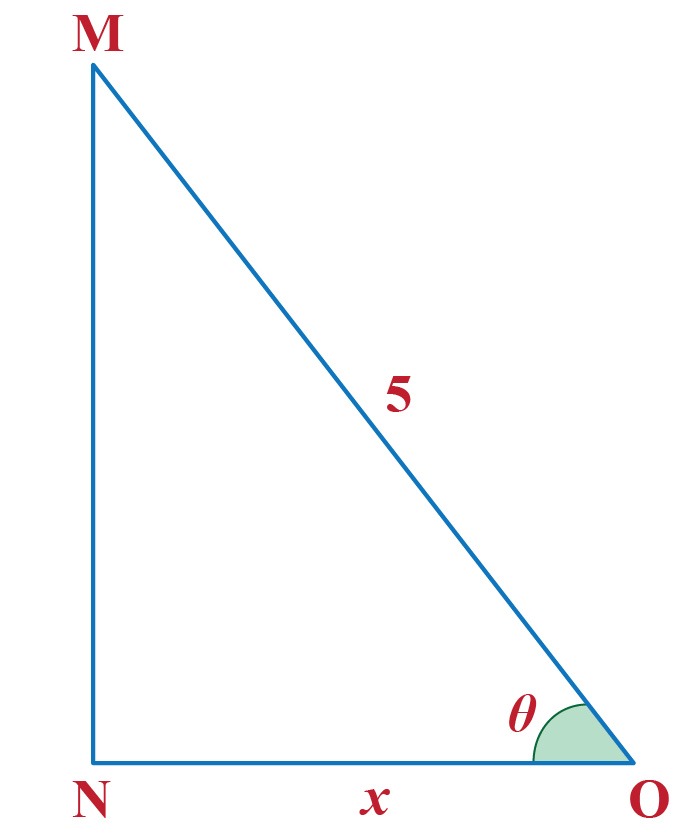Solution

Given,   $\cos \theta = 0.6$

Since,\begin{align*} \cos \theta &=\dfrac{\text {base}}{\text{Hypotenuse}} \\ \implies 0.6 &= \dfrac{\text {base}}{\text{hypotenuse}} \\ \implies 0.6 &= \dfrac x5 \\ \implies x &= 3\end{align*}

 $$\therefore$$ x = 3
 Example 3

Rayan wants to find the exact length of the shadow cast by a 15 ft lamp post when the angle of elevation of the sun is 60º.

Solution

Let us assume that the length of the shadow of the lamp post is $$x$$ ft.

Using the given information: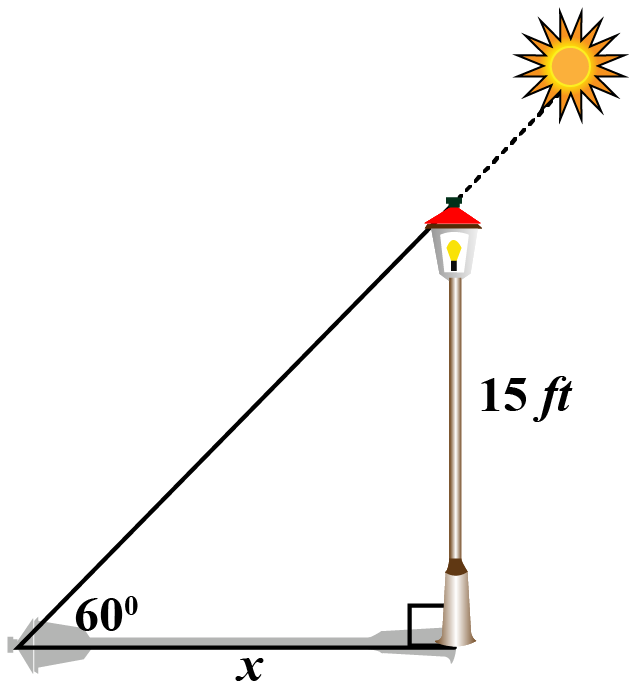Applying tan to the given triangle

\begin{align} \tan 60^\circ &= \dfrac{15}{x}\\[0.2cm] x&= \dfrac{15}{\tan 60^\circ}\\[0.2cm] x &= \dfrac{15}{\sqrt 3} \,\,\, \text{(Using chart)} \\[0.2cm] x&= \dfrac{15 \sqrt 3}{3}\end{align}

 $$\therefore$$ The length of the shadow of the lamp post is $$\dfrac{15 \sqrt 3}{3}$$ ft.Think Tank
1. What can you say about the symmetry of the graph of the sine function?
2. Is sine function an odd function or an even function?

## Interactive Questions

Select/Type your answer and click the "Check Answer" button to see the result.

## Let's Summarize

We hope you enjoyed learning about the cosine function with the simulations and practice questions. Now you will be able to easily solve problems on all the cosine functions and trigonometric ratios.

## About Cuemath

At Cuemath, our team of math experts is dedicated to making learning fun for our favorite readers, the students!

Through an interactive and engaging learning-teaching-learning approach, the teachers explore all angles of a topic.

Be it worksheets, online classes, doubt sessions, or any other form of relation, it’s the logical thinking and smart learning approach that we at Cuemath believe in.

## 1.What are the properties of sine and cosine functions?

The sine function is an odd function.
The cosine function is an even function.
Both sine and cosine functions are periodic having a period of $$2\pi$$.

## 2. What is the inverse of cos?

We denote the inverse function as $$\text y=\cos^{−1}(x)$$. It gives the angle whose cosine is a given number.

## 3. What is the inverse trigonometric function of cosine?

We denote the inverse function as $$\text y=\cos^{−1}(x)$$. It gives the angle whose cosine is a given number.

## 4. What are the 6 inverse trigonometric functions?

6 inverse trig functions are $$\sin^{−1}(x)$$, $$\cos^{−1}(x)$$, $$\tan^{−1}(x)$$, $$\cot^{−1}(x)$$, $$\sec^{−1}(x)$$, $$\text{cosec}^{−1}(x)$$.

## 5. What is the function of cosine?

The cosine function equal to the ratio of base and hypotenuse of a right triangle.

## 6. What does a cosine curve look like?

The cosine curve is an up-down curve that repeats after every $$2\pi$$ radians.

## 7. Where is cos defined?

Cos is defined for all values of real numbers.

## 8. Is cos an even function?

The cosine function is an even function because $$\cos(−x) = \cos x.$$

## 9. Which trigonometric functions are even?

Cosine and secant are the trigonometric functions that are even.

## 10. What is the formula for cos2x?

cos2x formula =  Cos2x – Sin2x = 2Cos2x – 1= 1 – 2sin2x

Download Trigonometry Worksheets
Trigonometry
Grade 10 | Answers Set 1
Trigonometry
Grade 9 | Questions Set 1
Trigonometry
Grade 10 | Questions Set 1
Trigonometry
Grade 9 | Answers Set 1
More Important Topics
Numbers
Algebra
Geometry
Measurement
Money
Data
Trigonometry
Calculus